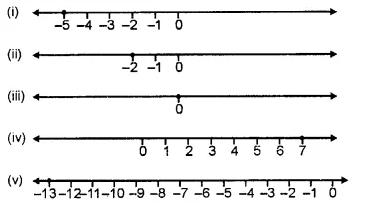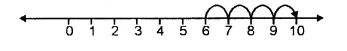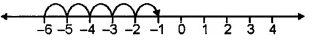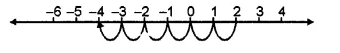## RS Aggarwal Class 6 Solutions Chapter 4 Integers Ex 4A

These Solutions are part of RS Aggarwal Solutions Class 6. Here we have given RS Aggarwal Solutions Class 6 Chapter 4 Integers Ex 4A.

Other Exercises

Question 1.
Solution:
(i) A decrease of 8
(ii) A gain of Rs. 7
(iii) Loosing a weight of 5 kg
(iv) 10 km below sea level
(v) 5°C above the freezing point
(vi) A withdrawal of Rs. 100
(vii) Spending Rs. 500
(viii) Going 6 m to the west
(ix) – 24
(x) 34

Question 2.
Solution:
(i) + Rs. 600
(ii) – Rs. 800
(iii) – 7°C
(iv) – 9
(v) + 2 km
(vi) – 3 km
(vii) + Rs. 200
(viii) – Rs. 300

Question 3.
Solution:Question 4.
Solution:
(i) 0
(ii) – 3
(iii) 2
(iv) 8
(v) – 365
(vi) 8

Question 5.
Solution:
(i) – 7
(ii) – 1
(iii) – 27
(iv) – 26
(v) – 603
(vi) – 777

Question 6.
Solution:
(i) The integers between 0 and 6 are
1, 2, 3, 4, 5.
(ii) The integers between – 5 and 0 are
– 4, – 3, – 2, – 1.
(iii) The integers between – 3 and 3 are
– 2, – 1, 0, 1, 2.
(iv) The integer between – 7 and – 5 is – 6.

Question 7.
Solution:
(i) 0 < 7
(ii) 0 > – 3
(iii) – 5 < – 2
(iv) – 15 < 13
(v) – 231 < – 132
(vi) – 6 < 6

Question 8.
Solution:
(i) – 7, – 2, 0, 5, 8
(ii) – 100, – 23, – 6, – 1, 0, 12
(iii) – 501, – 363, – 17, 15, 165
(iv) – 106, – 81, – 16, – 2, 0, 16, 21.

Question 9.
Solution:
(i) 36, 7, 0, – 3, – 9, – 132
(ii) 51, 0, – 2, – 8, – 53
(iii) 36, 0, – 5, – 71, – 81
(iv) 413, 102, – 7, – 365, – 515.

Question 10.
Solution:
(i) We want to write an integer 4 more than 6. So, we start from 6 and proceed 4 steps to the right to obtain 10, as shown below:∴ 4 more than 6 is 10.
(ii) We want to write an integer 5 more than – 6. So, we start from – 6 and proceed 5 steps to the right to obtain – 1, as shown below :∴ 5 more than – 6 is – 1.
(iii) We want to write an integer 6 less than 2. So we start from 2 and come back to the left by 6 steps to obtain – 4, as shown below:∴ 6 less than 2 is – 4.
(iv) We want to write an integer 2 less than – 3. So we start from – 3 and come back to the left by 2 steps to obtain – 5, as shown below :∴ 2 less than – 3 is – 5.

Question 11.
Solution:
(i) False, as zero is greater than every negative integer.
(ii) False, as zero is an integer.
(iii) True, as zero is neither positive nor negative.
(iv) False, as – 10 is to the left of – 6 on a number line.
(v) False, as absolute value of an integer is always equal to the integer.
(vi) True, as 0 is to right of every negative integer, on a number line.
(vii) False, as every natural number is positive. False, the successor is – 186
(viii) False, the predecessor is – 216

Question 12.
Solution:
(i) | – 9 | = 9
(ii) | 36 | = 36
(iii) | 0 | = 0
(iv) | 15 | = 15
(v) – | – 3 | = – 3
(vi) 7 + | – 3 | = 7 + 3 = 10
(vii) |7 – 4| = | 3 | = 3
(viii) 8 – | – 7| = 8 – 7 = 1

Question 13.
Solution:
The required integers are – 6, – 5, – 4, – 3, – 2.
The required integers are – 21, – 22, – 23, – 24, – 25.
The required integers are – 21, – 22, – 23, – 24, – 25.

Hope given RS Aggarwal Solutions Class 6 Chapter 4 Integers Ex 4A are helpful to complete your math homework.

If you have any doubts, please comment below. Learn Insta try to provide online math tutoring for you.